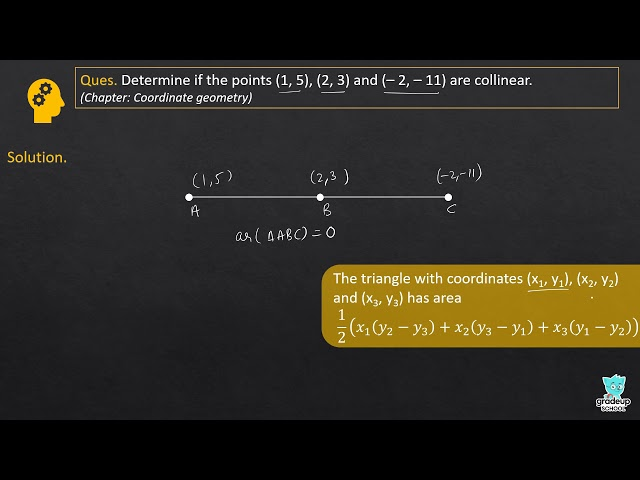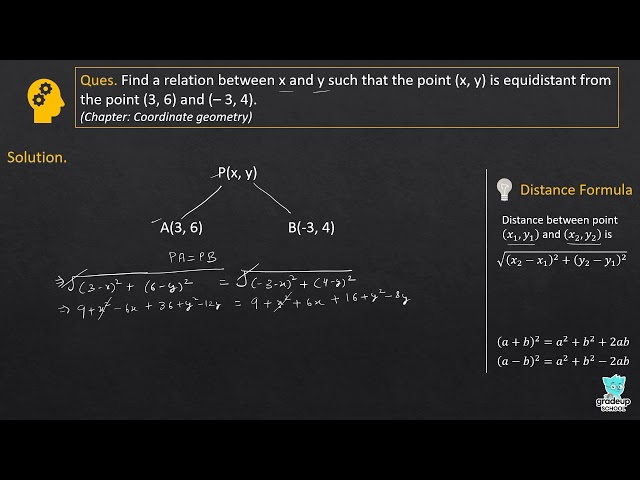# NCERT Solutions for Class 10 Maths Chapter 7 - Coordinate Geometry

Share

Goprep provides students with NCERT Mathematics Solutions for Class 10th Chapter 7- Coordinate Geometry to aid students in learning the concepts of the topics and solving the questions asked at the end of each chapter. These NCERT Solutions are drafted after extensive research and in sync with the official syllabus which is approved by CBSE. You will find these Solutions extremely helpful for the students in understanding the basics of the topic and solving the questions of the textbook in an efficient way.

The Chapter 7 - Coordinate Geometry deals with How to find the distance between two points whose coordinates are given, How to find the area of the Triangle formed by three given points, How to find the coordinates of the point which divides a line segment joining two given points in two ratios, Distance Formula, Section Formula, Area of a Triangle etc.

## NCERT Solutions for Class 10 Maths Chapter 7 - Coordinate Geometry

Exercise 7.1
• Exercise 7.1
• Exercise 7.2
• Exercise 7.3
• Exercise 7.4
Class 10th|NCERT - MathematicsChapter 7 - Coordinate GeometryNCERT - MathematicsClass 10th , NCERT
 Chapter 1 - Real Numbers Chapter 2 - Polynomials Chapter 3 - Pair of Linear Equations in Two Variables Chapter 4 - Quadratic Equations Chapter 5 - Arithmetic Progressions Chapter 6 - Triangles Chapter 7 - Coordinate Geometry Chapter 8 - Introduction to Trigonometry Chapter 9 - Some Applications of Trigonometry Chapter 10 - Circles Chapter 11 - Constructions Chapter 12 - Areas Related to Circles Chapter 13 - Surface Areas and Volumes Chapter 14 - Statistics Chapter 15 - Probability